# RD Sharma Solutions for Class 8 Maths Chapter 13 Profit, Loss, Discount and Value Added Tax (VAT) Exercise 13.1

In Exercise 13.1 of Chapter 13, we shall discuss more problems based on profit and loss including profit percentage and loss percentage. Students can refer to RD Sharma Solutions which is prepared by our expert tutors to help students clarify their doubts pertaining to this chapter. On regular practice, students can speed up the method of solving problems by using short-cut tips to secure high marks in their examination. Students can download the pdf easily from the links given below.

## Download the Pdf of RD Sharma Solutions for Class 8 Maths Exercise 13.1 Chapter 13 Profit, Loss, Discount and Value Added Tax (VAT)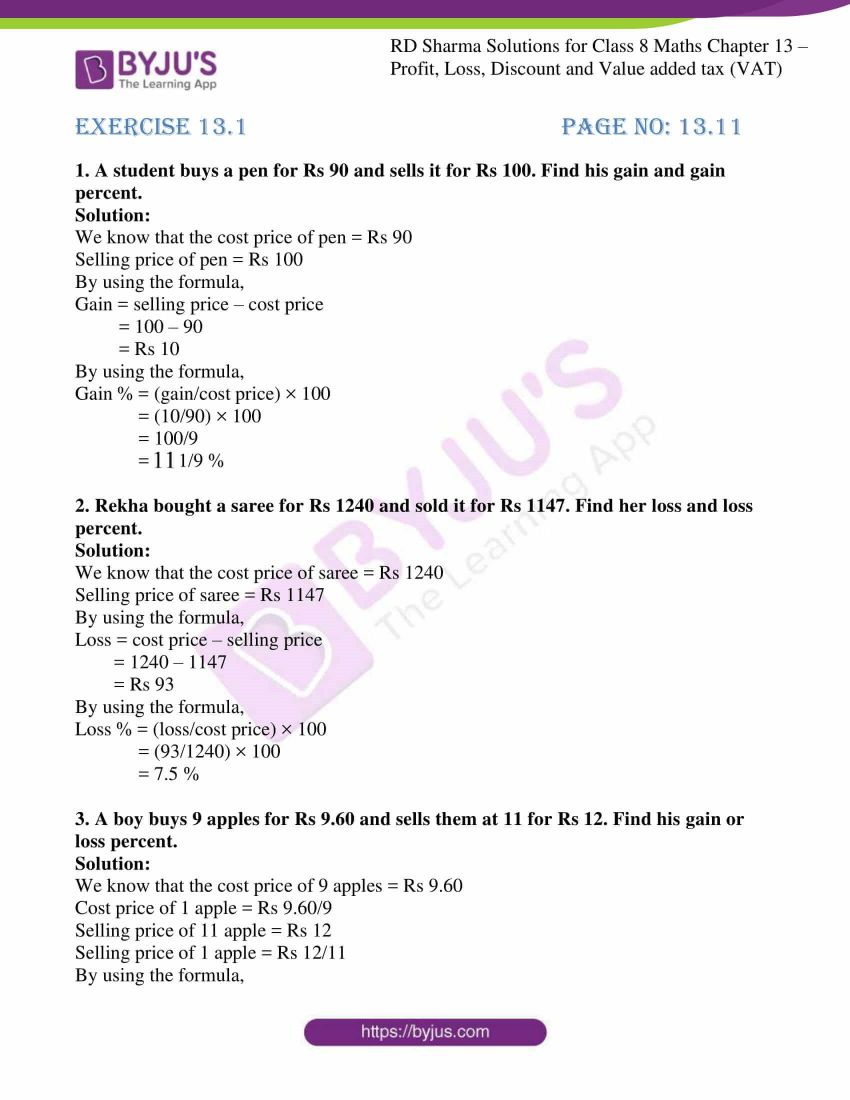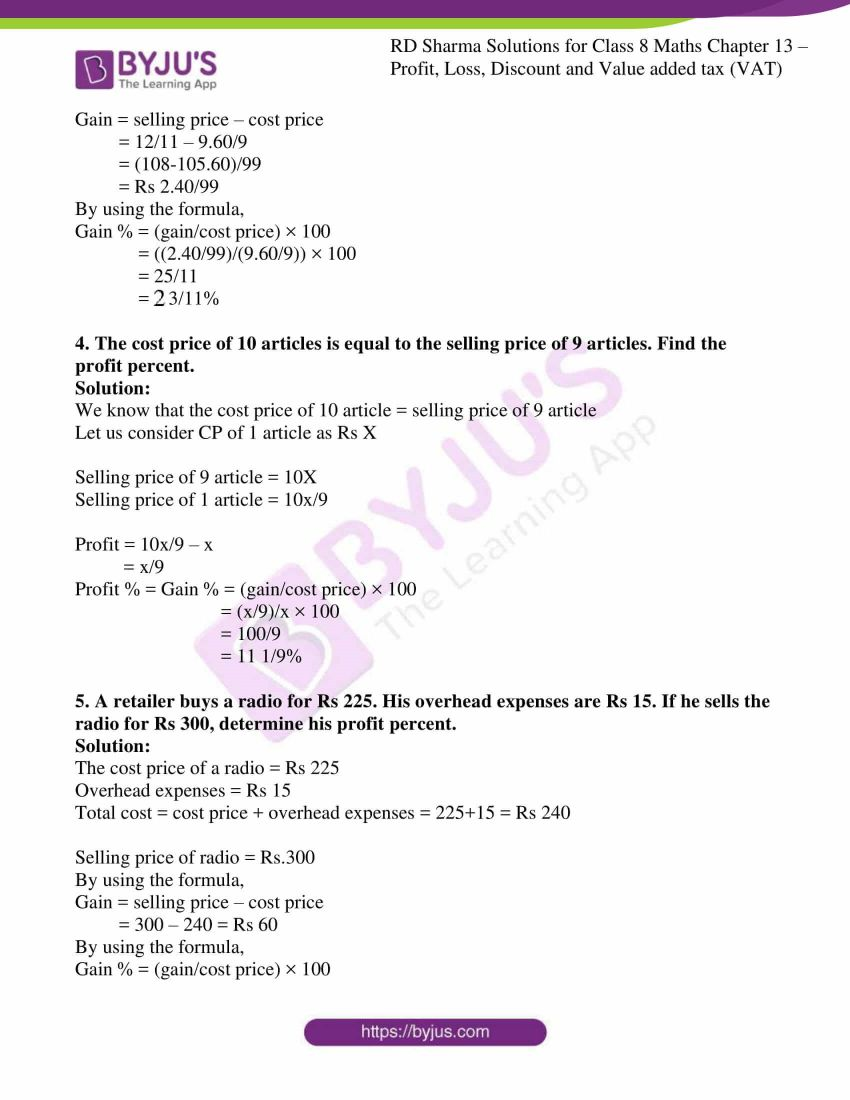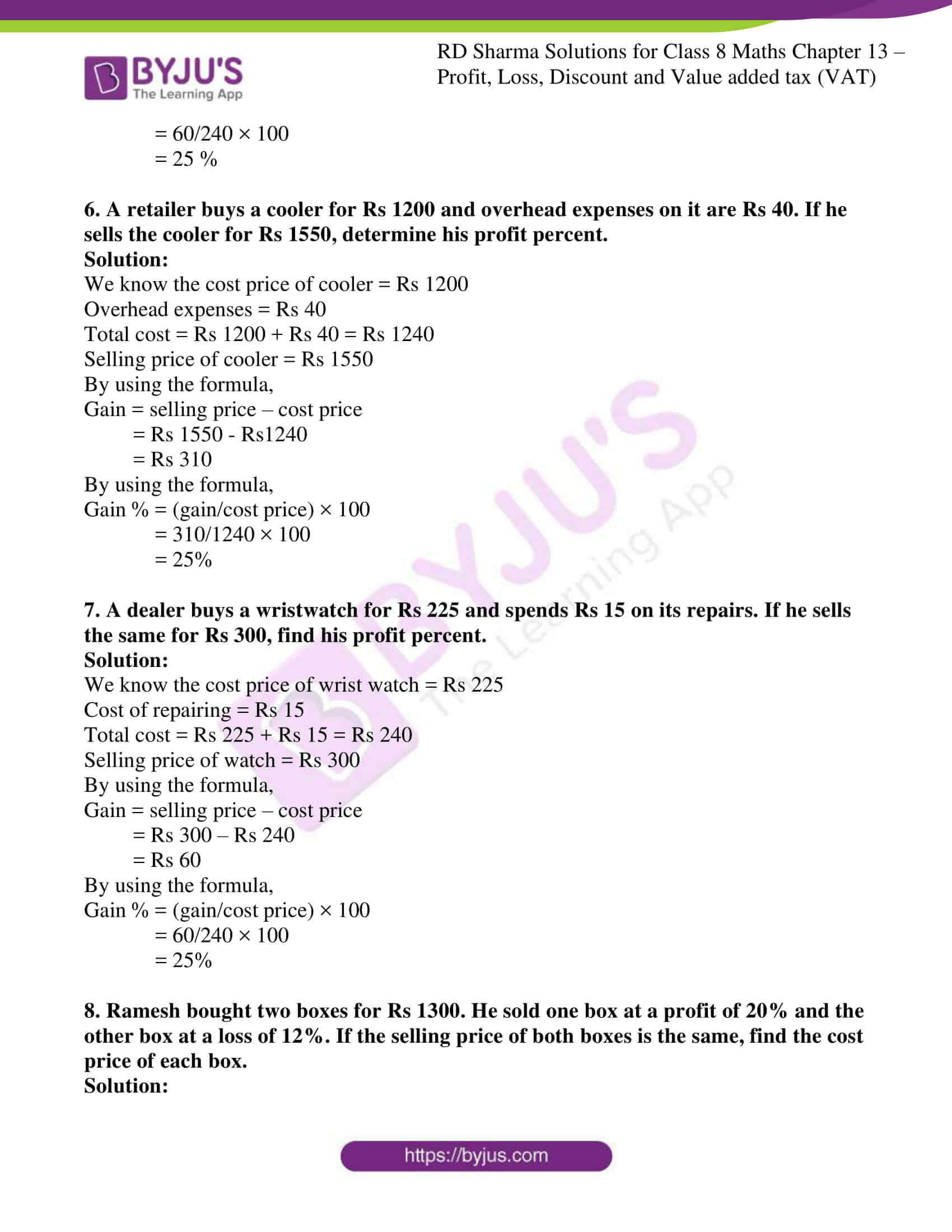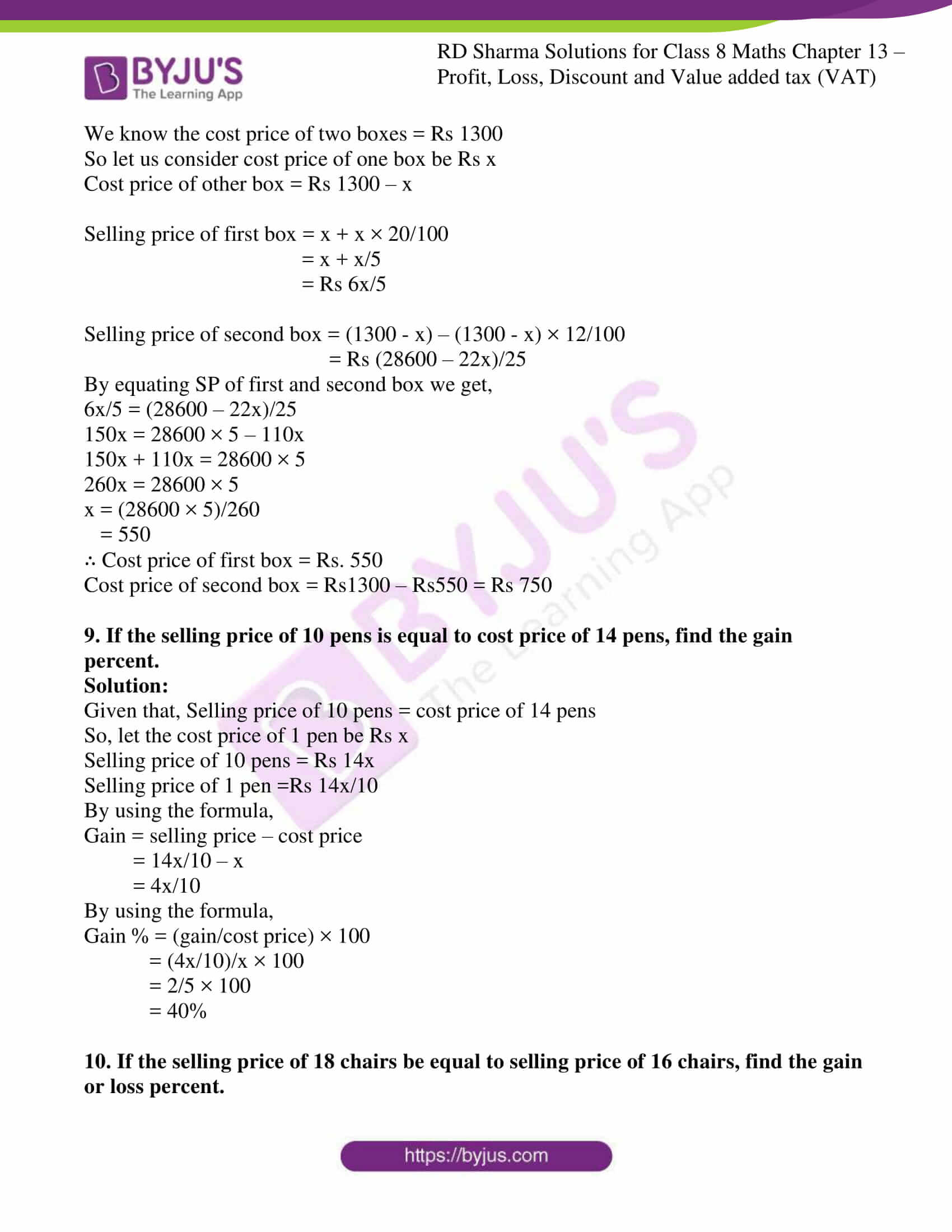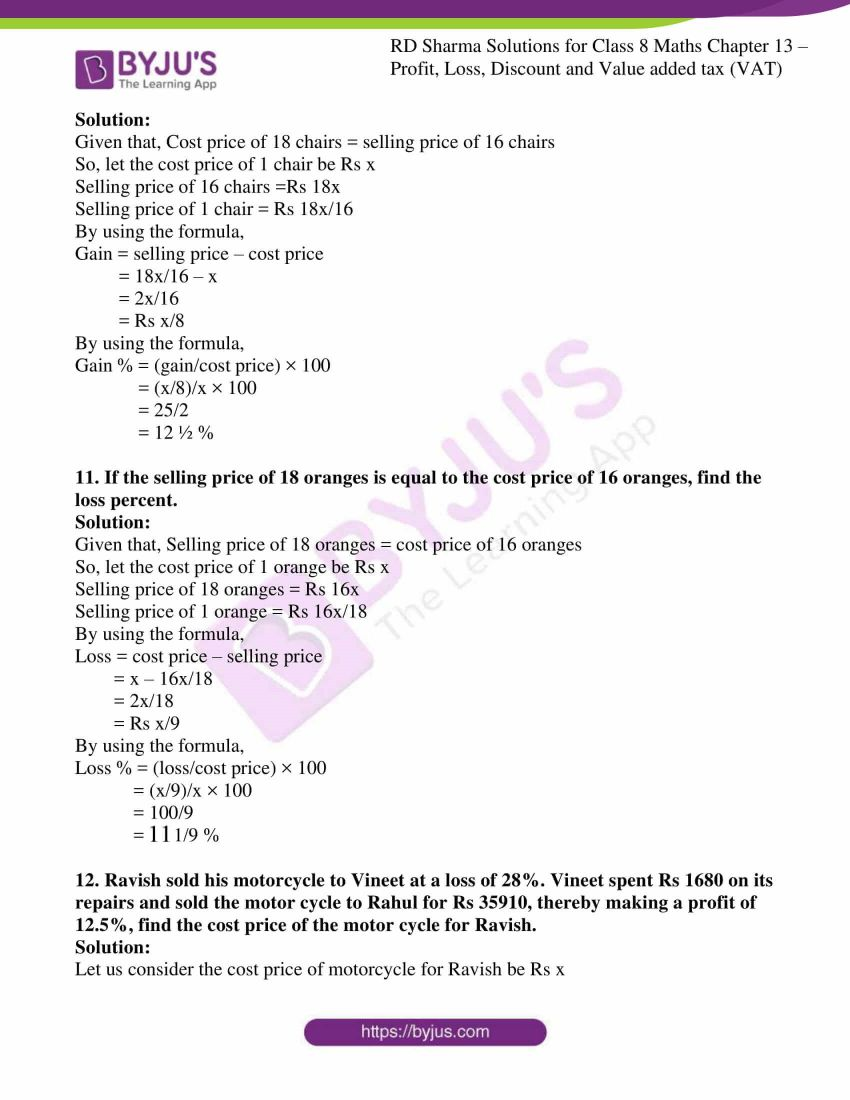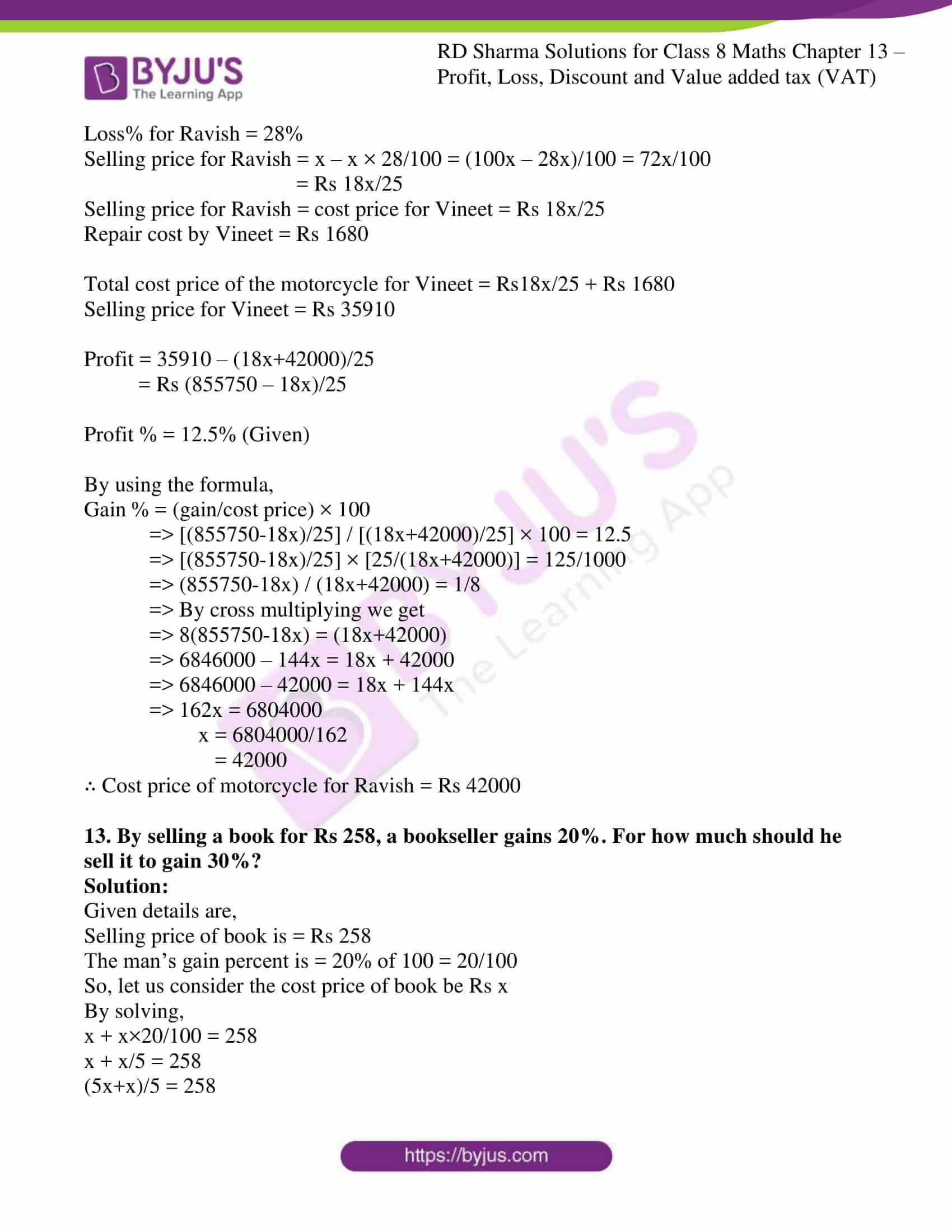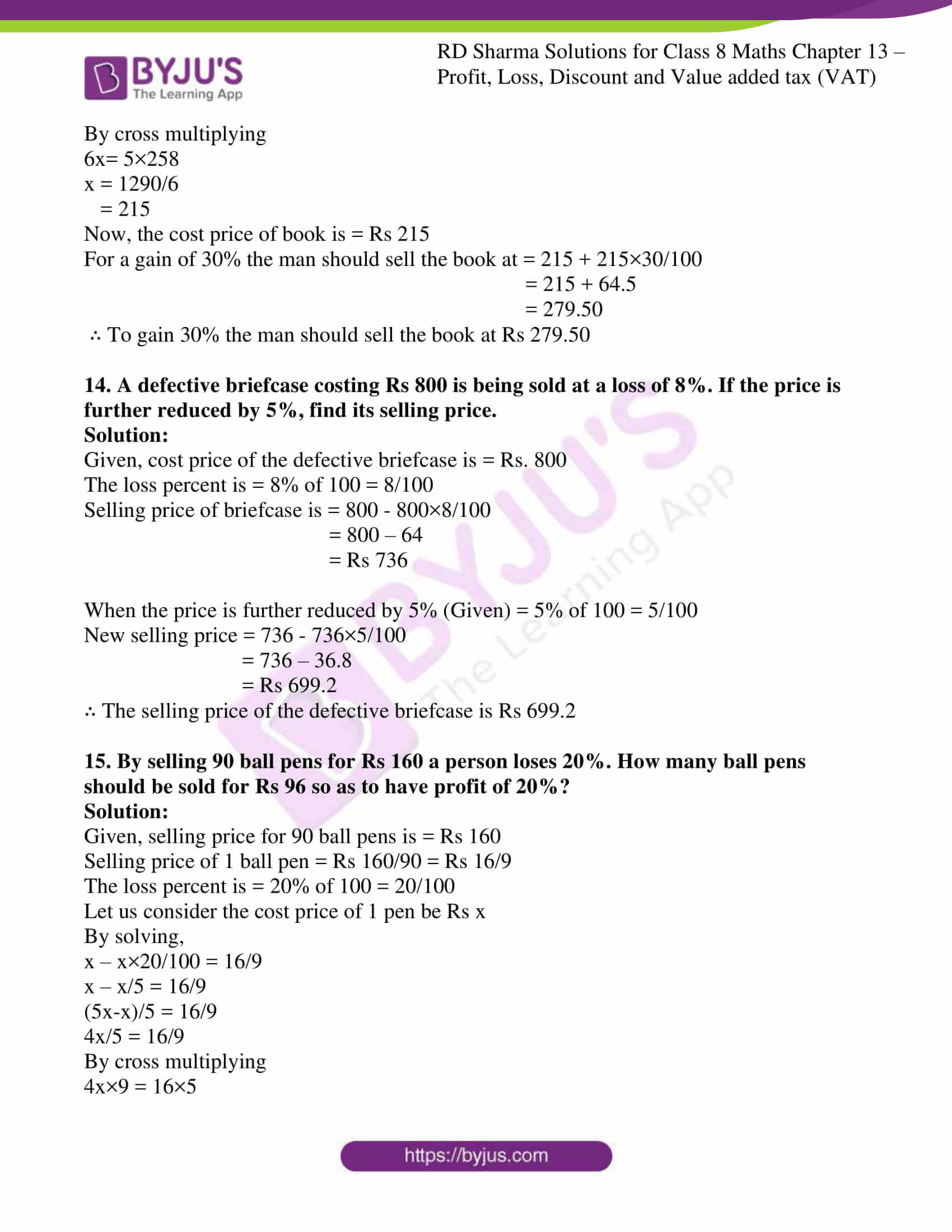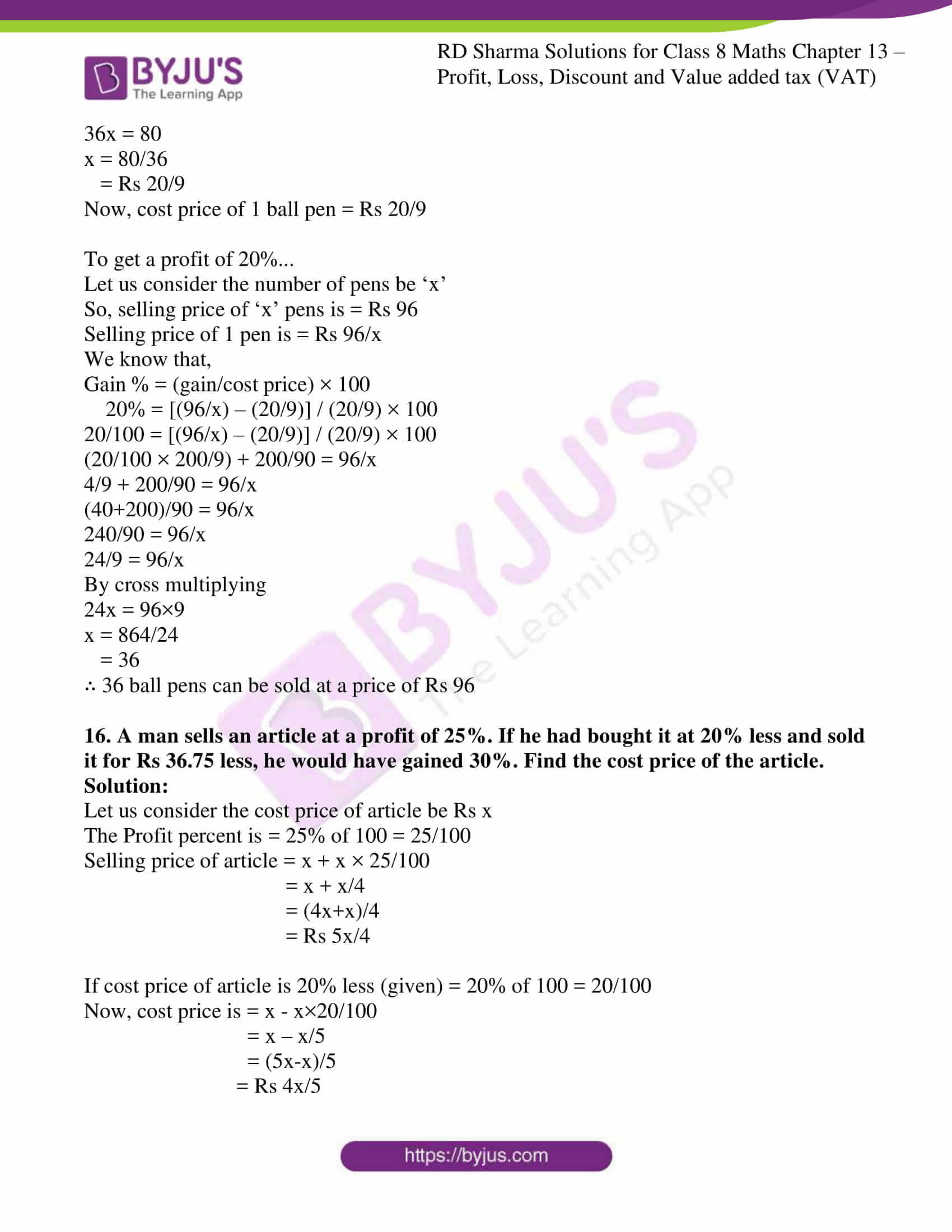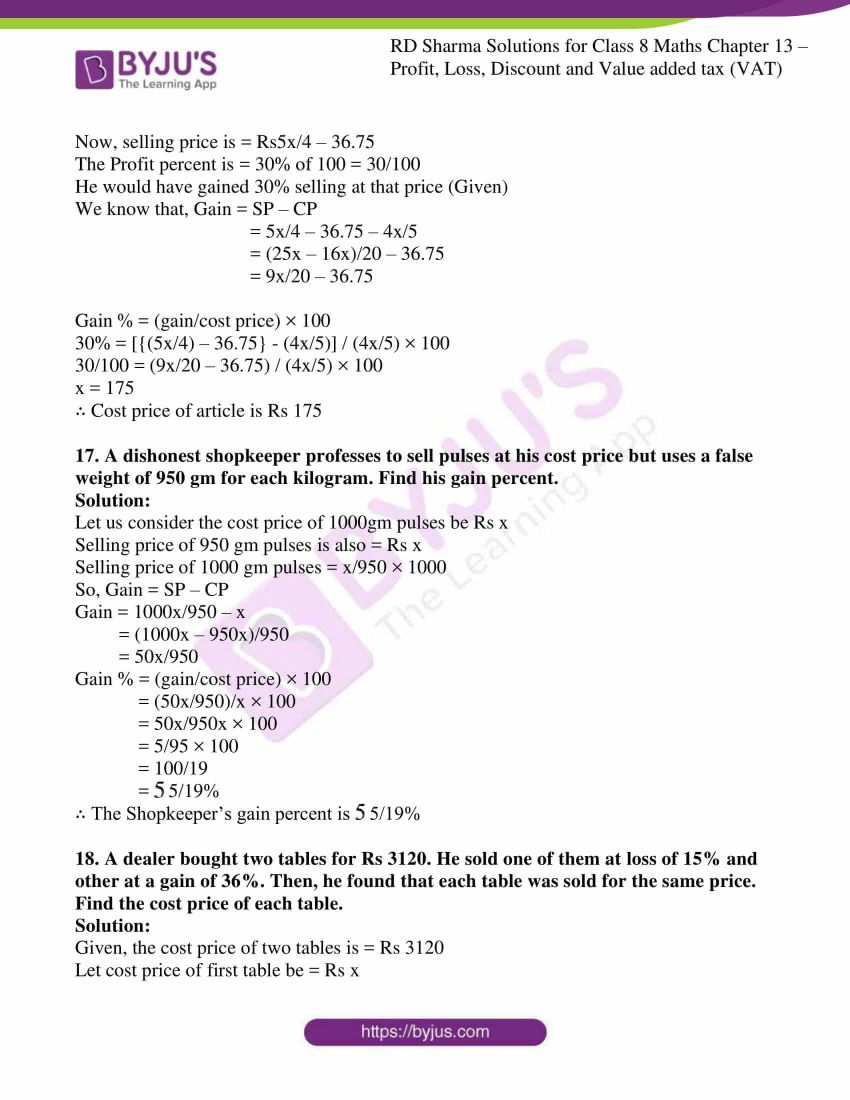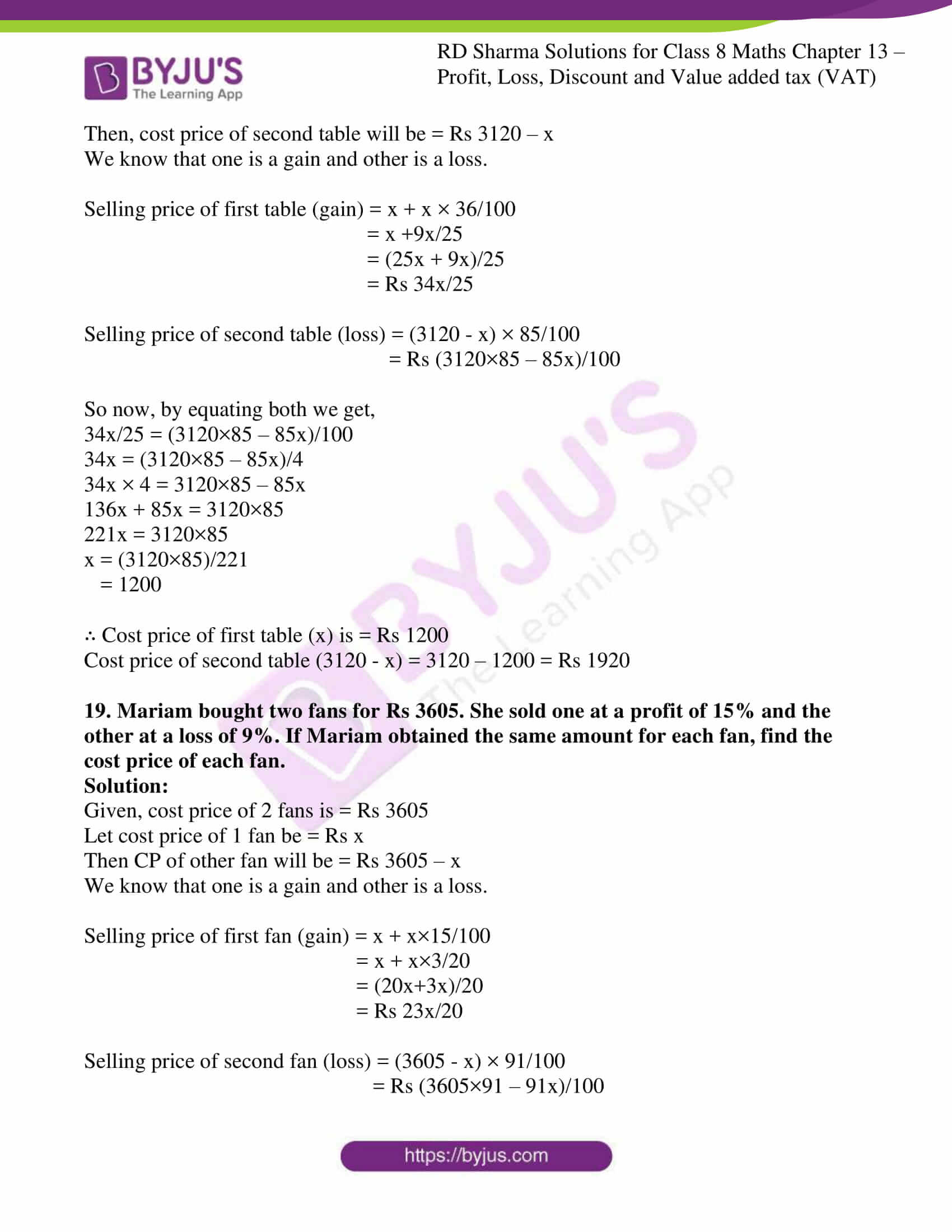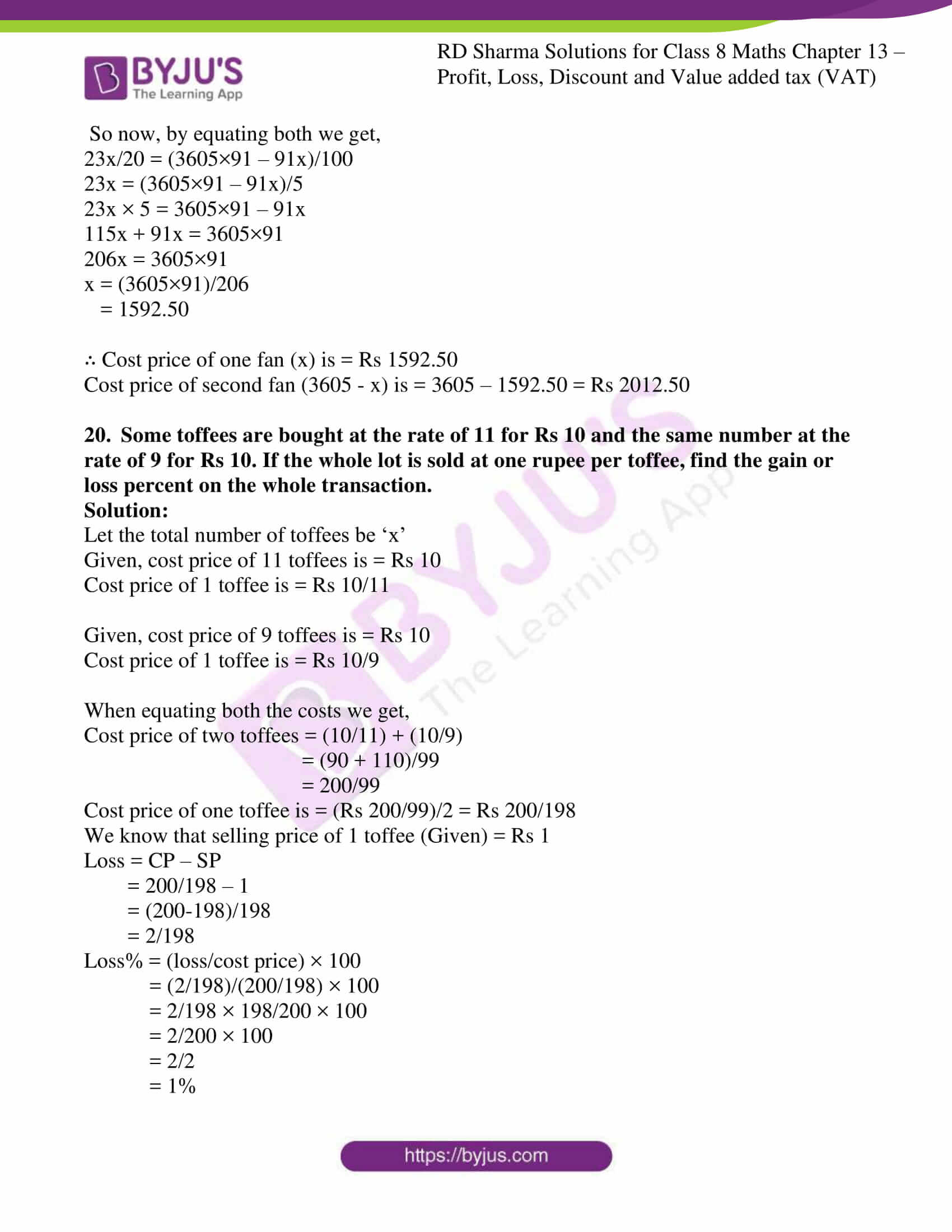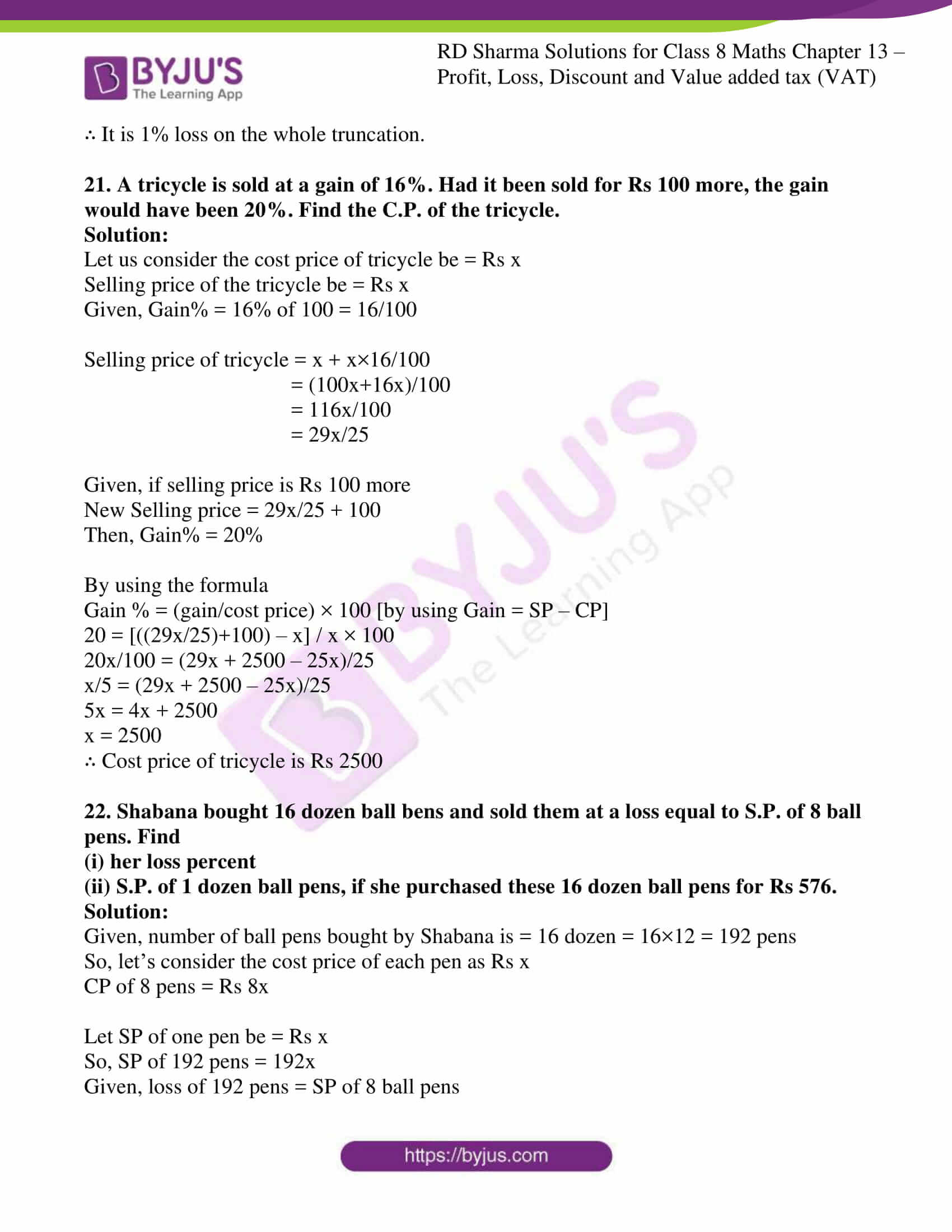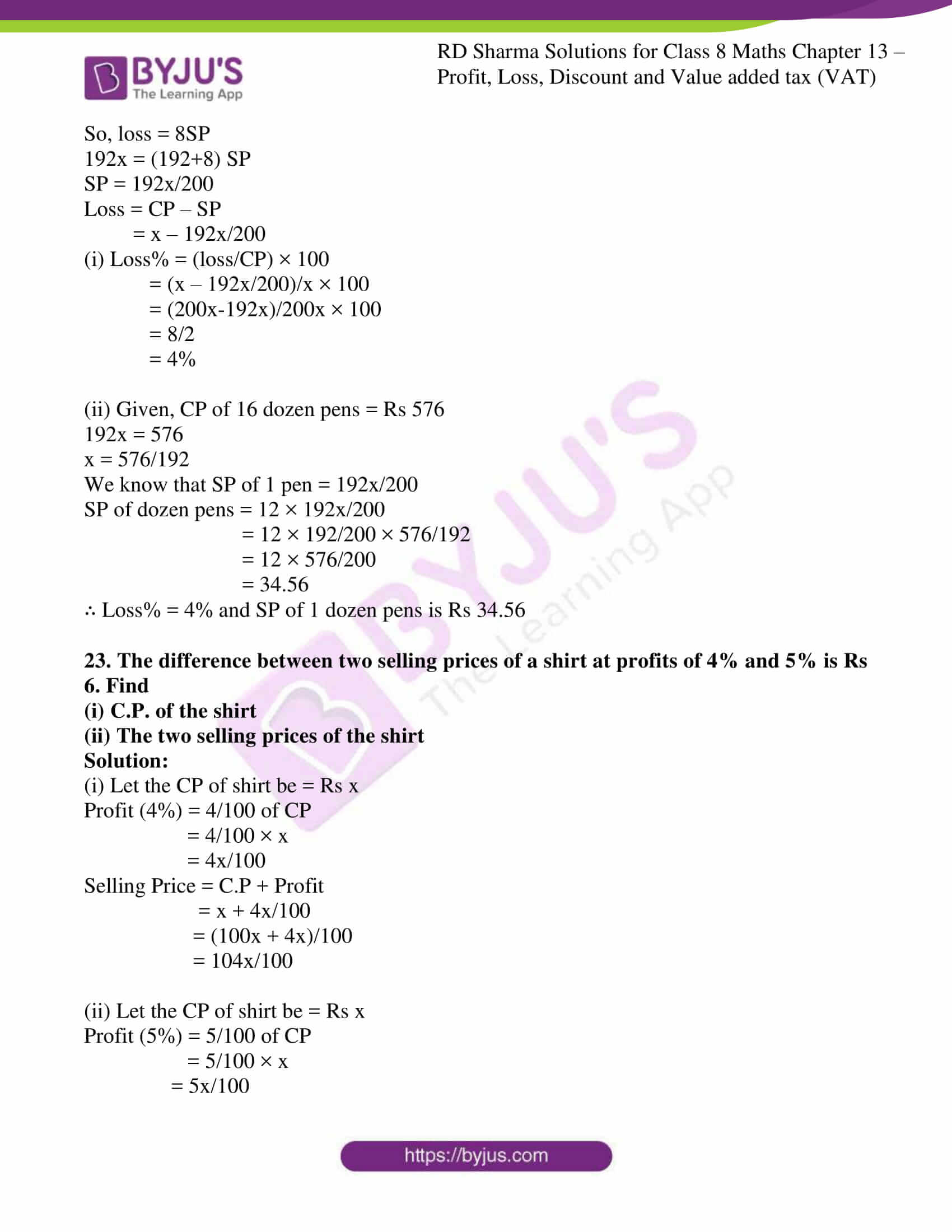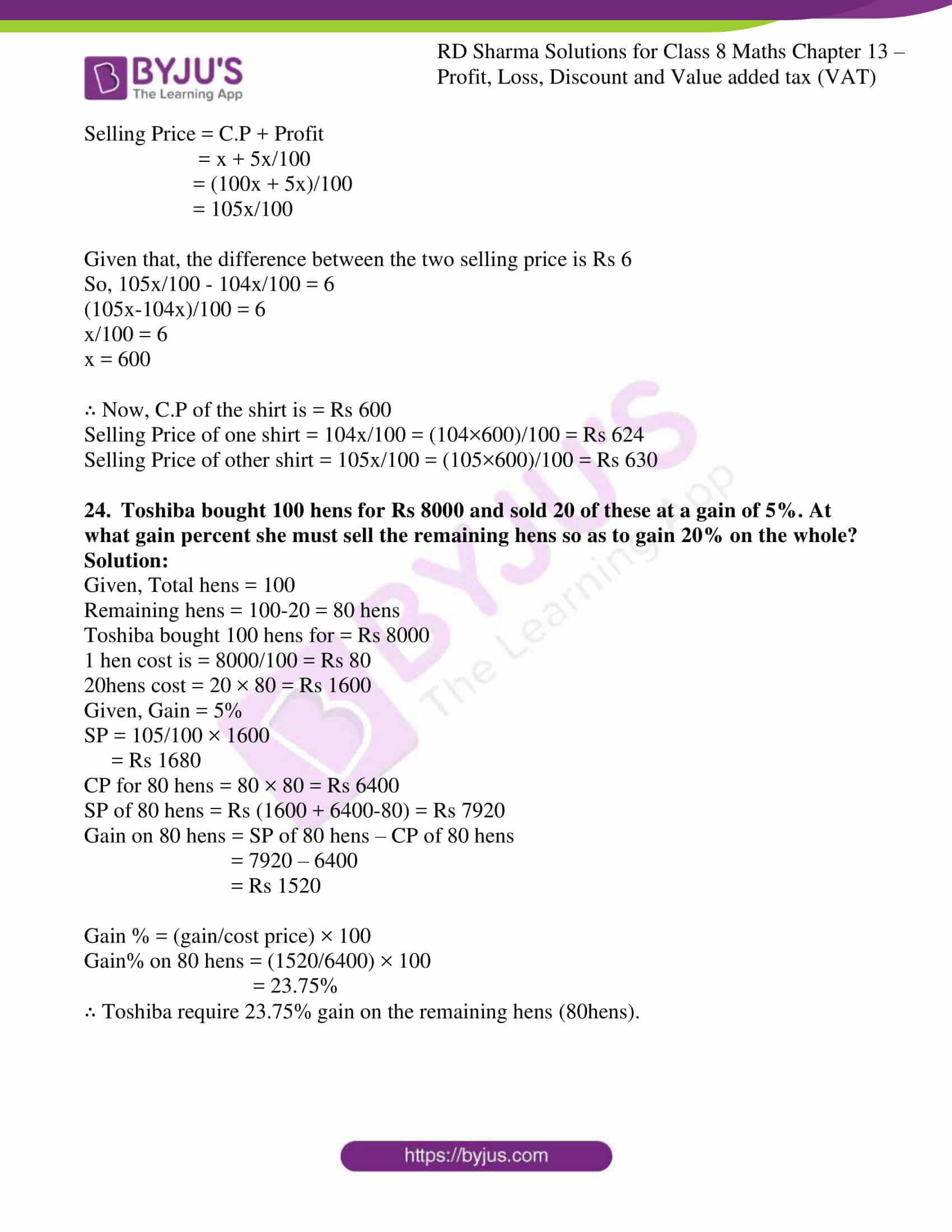### Access answers to Maths RD Sharma Solutions For Class 8 Exercise 13.1 Chapter 13 Profit, Loss, Discount and Value Added Tax (VAT)

1. A student buys a pen for Rs 90 and sells it for Rs 100. Find his gain and gain percent.

Solution:

We know that the cost price of pen = Rs 90

Selling price of pen = Rs 100

By using the formula,

Gain = selling price – cost price

= 100 – 90

= Rs 10

By using the formula,

Gain % = (gain/cost price) × 100

= (10/90) × 100

= 100/9

= $$11\frac{1}{9}$$ %

2. Rekha bought a saree for Rs 1240 and sold it for Rs 1147. Find her loss and loss percent.

Solution:

We know that the cost price of saree = Rs 1240

Selling price of saree = Rs 1147

By using the formula,

Loss = cost price – selling price

= 1240 – 1147

= Rs 93

By using the formula,

Loss % = (loss/cost price) × 100

= (93/1240) × 100

= 7.5 %

3. A boy buys 9 apples for Rs 9.60 and sells them at 11 for Rs 12. Find his gain or loss percent.

Solution:

We know that the cost price of 9 apples = Rs 9.60

Cost price of 1 apple = Rs 9.60/9

Selling price of 11 apple = Rs 12

Selling price of 1 apple = Rs 12/11

By using the formula,

Gain = selling price – cost price

= 12/11 – 9.60/9

= (108-105.60)/99

= Rs 2.40/99

By using the formula,

Gain % = (gain/cost price) × 100

= ((2.40/99)/(9.60/9)) × 100

= 25/11

= $$2\frac{3}{11}$$%

4. The cost price of 10 articles is equal to the selling price of 9 articles. Find the profit percent.

Solution:

We know that the cost price of 10 article = selling price of 9 article

Let us consider CP of 1 article as Rs X

Selling price of 9 article = 10X

Selling price of 1 article = 10x/9

Profit = 10x/9 – x

= x/9

Profit % = Gain % = (gain/cost price) × 100

= (x/9)/x × 100

= 100/9

= $$11\frac{1}{9}$$%

5. A retailer buys a radio for Rs 225. His overhead expenses are Rs 15. If he sells the radio for Rs 300, determine his profit percent.

Solution:

The cost price of a radio = Rs 225

Total cost = cost price + overhead expenses = 225+15 = Rs 240

Selling price of radio = Rs.300

By using the formula,

Gain = selling price – cost price

= 300 – 240 = Rs 60

By using the formula,

Gain % = (gain/cost price) × 100

= 60/240 × 100

= 25 %

6. A retailer buys a cooler for Rs 1200 and overhead expenses on it are Rs 40. If he sells the cooler for Rs 1550, determine his profit percent.

Solution:

We know the cost price of cooler = Rs 1200

Total cost = Rs 1200 + Rs 40 = Rs 1240

Selling price of cooler = Rs 1550

By using the formula,

Gain = selling price – cost price

= Rs 1550 – Rs1240

= Rs 310

By using the formula,

Gain % = (gain/cost price) × 100

= 310/1240 × 100

= 25%

7. A dealer buys a wristwatch for Rs 225 and spends Rs 15 on its repairs. If he sells the same for Rs 300, find his profit percent.

Solution:

We know the cost price of wrist watch = Rs 225

Cost of repairing = Rs 15

Total cost = Rs 225 + Rs 15 = Rs 240

Selling price of watch = Rs 300

By using the formula,

Gain = selling price – cost price

= Rs 300 – Rs 240

= Rs 60

By using the formula,

Gain % = (gain/cost price) × 100

= 60/240 × 100

= 25%

8. Ramesh bought two boxes for Rs 1300. He sold one box at a profit of 20% and the other box at a loss of 12%. If the selling price of both boxes is the same, find the cost price of each box.

Solution:

We know the cost price of two boxes = Rs 1300

So let us consider cost price of one box be Rs x

Cost price of other box = Rs 1300 – x

Selling price of first box = x + x × 20/100

= x + x/5

= Rs 6x/5

Selling price of second box = (1300 – x) – (1300 – x) × 12/100

= Rs (28600 – 22x)/25

By equating SP of first and second box we get,

6x/5 = (28600 – 22x)/25

150x = 28600 × 5 – 110x

150x + 110x = 28600 × 5

260x = 28600 × 5

x = (28600 × 5)/260

= 550

∴ Cost price of first box = Rs. 550

Cost price of second box = Rs1300 – Rs550 = Rs 750

9. If the selling price of 10 pens is equal to cost price of 14 pens, find the gain percent.

Solution:

Given that, Selling price of 10 pens = cost price of 14 pens

So, let the cost price of 1 pen be Rs x

Selling price of 10 pens = Rs 14x

Selling price of 1 pen =Rs 14x/10

By using the formula,

Gain = selling price – cost price

= 14x/10 – x

= 4x/10

By using the formula,

Gain % = (gain/cost price) × 100

= (4x/10)/x × 100

= 2/5 × 100

= 40%

10. If the selling price of 18 chairs be equal to selling price of 16 chairs, find the gain or loss percent.

Solution:

Given that, Cost price of 18 chairs = selling price of 16 chairs

So, let the cost price of 1 chair be Rs x

Selling price of 16 chairs =Rs 18x

Selling price of 1 chair = Rs 18x/16

By using the formula,

Gain = selling price – cost price

= 18x/16 – x

= 2x/16

= Rs x/8

By using the formula,

Gain % = (gain/cost price) × 100

= (x/8)/x × 100

= 25/2

= 12 ½ %

11. If the selling price of 18 oranges is equal to the cost price of 16 oranges, find the loss percent.

Solution:

Given that, Selling price of 18 oranges = cost price of 16 oranges

So, let the cost price of 1 orange be Rs x

Selling price of 18 oranges = Rs 16x

Selling price of 1 orange = Rs 16x/18

By using the formula,

Loss = cost price – selling price

= x – 16x/18

= 2x/18

= Rs x/9

By using the formula,

Loss % = (loss/cost price) × 100

= (x/9)/x × 100

= 100/9

= $$11\frac{1}{9}$$ %

12. Ravish sold his motorcycle to Vineet at a loss of 28%. Vineet spent Rs 1680 on its repairs and sold the motor cycle to Rahul for Rs 35910, thereby making a profit of 12.5%, find the cost price of the motor cycle for Ravish.

Solution:

Let us consider the cost price of motorcycle for Ravish be Rs x

Loss% for Ravish = 28%

Selling price for Ravish = x – x × 28/100 = (100x – 28x)/100 = 72x/100

= Rs 18x/25

Selling price for Ravish = cost price for Vineet = Rs 18x/25

Repair cost by Vineet = Rs 1680

Total cost price of the motorcycle for Vineet = Rs18x/25 + Rs 1680

Selling price for Vineet = Rs 35910

Profit = 35910 – (18x+42000)/25

= Rs (855750 – 18x)/25

Profit % = 12.5% (Given)

By using the formula,

Gain % = (gain/cost price) × 100

=> [(855750-18x)/25] / [(18x+42000)/25] × 100 = 12.5

=> [(855750-18x)/25] × [25/(18x+42000)] = 125/1000

=> (855750-18x) / (18x+42000) = 1/8

=> By cross multiplying we get

=> 8(855750-18x) = (18x+42000)

=> 6846000 – 144x = 18x + 42000

=> 6846000 – 42000 = 18x + 144x

=> 162x = 6804000

x = 6804000/162

= 42000

∴ Cost price of motorcycle for Ravish = Rs 42000

13. By selling a book for Rs 258, a bookseller gains 20%. For how much should he sell it to gain 30%?

Solution:

Given details are,

Selling price of book is = Rs 258

The man’s gain percent is = 20% of 100 = 20/100

So, let us consider the cost price of book be Rs x

By solving,

x + x×20/100 = 258

x + x/5 = 258

(5x+x)/5 = 258

By cross multiplying

6x= 5×258

x = 1290/6

= 215

Now, the cost price of book is = Rs 215

For a gain of 30% the man should sell the book at = 215 + 215×30/100

= 215 + 64.5

= 279.50

∴ To gain 30% the man should sell the book at Rs 279.50

14. A defective briefcase costing Rs 800 is being sold at a loss of 8%. If the price is further reduced by 5%, find its selling price.

Solution:

Given, cost price of the defective briefcase is = Rs. 800

The loss percent is = 8% of 100 = 8/100

Selling price of briefcase is = 800 – 800×8/100

= 800 – 64

= Rs 736

When the price is further reduced by 5% (Given) = 5% of 100 = 5/100

New selling price = 736 – 736×5/100

= 736 – 36.8

= Rs 699.2

∴ The selling price of the defective briefcase is Rs 699.2

15. By selling 90 ball pens for Rs 160 a person loses 20%. How many ball pens should be sold for Rs 96 so as to have profit of 20%?

Solution:

Given, selling price for 90 ball pens is = Rs 160

Selling price of 1 ball pen = Rs 160/90 = Rs 16/9

The loss percent is = 20% of 100 = 20/100

Let us consider the cost price of 1 pen be Rs x

By solving,

x – x×20/100 = 16/9

x – x/5 = 16/9

(5x-x)/5 = 16/9

4x/5 = 16/9

By cross multiplying

4x×9 = 16×5

36x = 80

x = 80/36

= Rs 20/9

Now, cost price of 1 ball pen = Rs 20/9

To get a profit of 20%…

Let us consider the number of pens be ‘x’

So, selling price of ‘x’ pens is = Rs 96

Selling price of 1 pen is = Rs 96/x

We know that,

Gain % = (gain/cost price) × 100

20% = [(96/x) – (20/9)] / (20/9) × 100

20/100 = [(96/x) – (20/9)] / (20/9) × 100

(20/100 × 200/9) + 200/90 = 96/x

4/9 + 200/90 = 96/x

(40+200)/90 = 96/x

240/90 = 96/x

24/9 = 96/x

By cross multiplying

24x = 96×9

x = 864/24

= 36

∴ 36 ball pens can be sold at a price of Rs 96

16. A man sells an article at a profit of 25%. If he had bought it at 20% less and sold it for Rs 36.75 less, he would have gained 30%. Find the cost price of the article.

Solution:

Let us consider the cost price of article be Rs x

The Profit percent is = 25% of 100 = 25/100

Selling price of article = x + x × 25/100

= x + x/4

= (4x+x)/4

= Rs 5x/4

If cost price of article is 20% less (given) = 20% of 100 = 20/100

Now, cost price is = x – x×20/100

= x – x/5

= (5x-x)/5

= Rs 4x/5

Now, selling price is = Rs5x/4 – 36.75

The Profit percent is = 30% of 100 = 30/100

He would have gained 30% selling at that price (Given)

We know that, Gain = SP – CP

= 5x/4 – 36.75 – 4x/5

= (25x – 16x)/20 – 36.75

= 9x/20 – 36.75

Gain % = (gain/cost price) × 100

30% = [{(5x/4) – 36.75} – (4x/5)] / (4x/5) × 100

30/100 = (9x/20 – 36.75) / (4x/5) × 100

x = 175

∴ Cost price of article is Rs 175

17. A dishonest shopkeeper professes to sell pulses at his cost price but uses a false weight of 950 gm for each kilogram. Find his gain percent.

Solution:

Let us consider the cost price of 1000gm pulses be Rs x

Selling price of 950 gm pulses is also = Rs x

Selling price of 1000 gm pulses = x/950 × 1000

So, Gain = SP – CP

Gain = 1000x/950 – x

= (1000x – 950x)/950

= 50x/950

Gain % = (gain/cost price) × 100

= (50x/950)/x × 100

= 50x/950x × 100

= 5/95 × 100

= 100/19

= $$5\frac{5}{19}$$%

∴ The Shopkeeper’s gain percent is $$5\frac{5}{19}$$%

18. A dealer bought two tables for Rs 3120. He sold one of them at loss of 15% and other at a gain of 36%. Then, he found that each table was sold for the same price. Find the cost price of each table.

Solution:

Given, the cost price of two tables is = Rs 3120

Let cost price of first table be = Rs x

Then, cost price of second table will be = Rs 3120 – x

We know that one is a gain and other is a loss.

Selling price of first table (gain) = x + x × 36/100

= x +9x/25

= (25x + 9x)/25

= Rs 34x/25

Selling price of second table (loss) = (3120 – x) × 85/100

= Rs (3120×85 – 85x)/100

So now, by equating both we get,

34x/25 = (3120×85 – 85x)/100

34x = (3120×85 – 85x)/4

34x × 4 = 3120×85 – 85x

136x + 85x = 3120×85

221x = 3120×85

x = (3120×85)/221

= 1200

∴ Cost price of first table (x) is = Rs 1200

Cost price of second table (3120 – x) = 3120 – 1200 = Rs 1920

19. Mariam bought two fans for Rs 3605. She sold one at a profit of 15% and the other at a loss of 9%. If Mariam obtained the same amount for each fan, find the cost price of each fan.

Solution:

Given, cost price of 2 fans is = Rs 3605

Let cost price of 1 fan be = Rs x

Then CP of other fan will be = Rs 3605 – x

We know that one is a gain and other is a loss.

Selling price of first fan (gain) = x + x×15/100

= x + x×3/20

= (20x+3x)/20

= Rs 23x/20

Selling price of second fan (loss) = (3605 – x) × 91/100

= Rs (3605×91 – 91x)/100

So now, by equating both we get,

23x/20 = (3605×91 – 91x)/100

23x = (3605×91 – 91x)/5

23x × 5 = 3605×91 – 91x

115x + 91x = 3605×91

206x = 3605×91

x = (3605×91)/206

= 1592.50

∴ Cost price of one fan (x) is = Rs 1592.50

Cost price of second fan (3605 – x) is = 3605 – 1592.50 = Rs 2012.50

20. Some toffees are bought at the rate of 11 for Rs 10 and the same number at the rate of 9 for Rs 10. If the whole lot is sold at one rupee per toffee, find the gain or loss percent on the whole transaction.

Solution:

Let the total number of toffees be ‘x’

Given, cost price of 11 toffees is = Rs 10

Cost price of 1 toffee is = Rs 10/11

Given, cost price of 9 toffees is = Rs 10

Cost price of 1 toffee is = Rs 10/9

When equating both the costs we get,

Cost price of two toffees = (10/11) + (10/9)

= (90 + 110)/99

= 200/99

Cost price of one toffee is = (Rs 200/99)/2 = Rs 200/198

We know that selling price of 1 toffee (Given) = Rs 1

Loss = CP – SP

= 200/198 – 1

= (200-198)/198

= 2/198

Loss% = (loss/cost price) × 100

= (2/198)/(200/198) × 100

= 2/198 × 198/200 × 100

= 2/200 × 100

= 2/2

= 1%

∴ It is 1% loss on the whole truncation.

21. A tricycle is sold at a gain of 16%. Had it been sold for Rs 100 more, the gain would have been 20%. Find the C.P. of the tricycle.

Solution:

Let us consider the cost price of tricycle be = Rs x

Selling price of the tricycle be = Rs x

Given, Gain% = 16% of 100 = 16/100

Selling price of tricycle = x + x×16/100

= (100x+16x)/100

= 116x/100

= 29x/25

Given, if selling price is Rs 100 more

New Selling price = 29x/25 + 100

Then, Gain% = 20%

By using the formula

Gain % = (gain/cost price) × 100 [by using Gain = SP – CP]

20 = [((29x/25)+100) – x] / x × 100

20x/100 = (29x + 2500 – 25x)/25

x/5 = (29x + 2500 – 25x)/25

5x = 4x + 2500

x = 2500

∴ Cost price of tricycle is Rs 2500

22. Shabana bought 16 dozen ball bens and sold them at a loss equal to S.P. of 8 ball pens. Find
(i) her loss percent
(ii) S.P. of 1 dozen ball pens, if she purchased these 16 dozen ball pens for Rs 576.

Solution:

Given, number of ball pens bought by Shabana is = 16 dozen = 16×12 = 192 pens

So, let’s consider the cost price of each pen as Rs x

CP of 8 pens = Rs 8x

Let SP of one pen be = Rs x

So, SP of 192 pens = 192x

Given, loss of 192 pens = SP of 8 ball pens

So, loss = 8SP

192x = (192+8) SP

SP = 192x/200

Loss = CP – SP

= x – 192x/200

(i) Loss% = (loss/CP) × 100

= (x – 192x/200)/x × 100

= (200x-192x)/200x × 100

= 8/2

= 4%

(ii) Given, CP of 16 dozen pens = Rs 576

192x = 576

x = 576/192

We know that SP of 1 pen = 192x/200

SP of dozen pens = 12 × 192x/200

= 12 × 192/200 × 576/192

= 12 × 576/200

= 34.56

∴ Loss% = 4% and SP of 1 dozen pens is Rs 34.56

23. The difference between two selling prices of a shirt at profits of 4% and 5% is Rs 6. Find
(i) C.P. of the shirt
(ii) The two selling prices of the shirt

Solution:

(i) Let the CP of shirt be = Rs x

Profit (4%) = 4/100 of CP

= 4/100 × x

= 4x/100

Selling Price = C.P + Profit

= x + 4x/100

= (100x + 4x)/100

= 104x/100

(ii) Let the CP of shirt be = Rs x

Profit (5%) = 5/100 of CP

= 5/100 × x

= 5x/100

Selling Price = C.P + Profit

= x + 5x/100

= (100x + 5x)/100

= 105x/100

Given that, the difference between the two selling price is Rs 6

So, 105x/100 – 104x/100 = 6

(105x-104x)/100 = 6

x/100 = 6

x = 600

∴ Now, C.P of the shirt is = Rs 600

Selling Price of one shirt = 104x/100 = (104×600)/100 = Rs 624

Selling Price of other shirt = 105x/100 = (105×600)/100 = Rs 630

24. Toshiba bought 100 hens for Rs 8000 and sold 20 of these at a gain of 5%. At what gain percent she must sell the remaining hens so as to gain 20% on the whole?

Solution:

Given, Total hens = 100

Remaining hens = 100-20 = 80 hens

Toshiba bought 100 hens for = Rs 8000

1 hen cost is = 8000/100 = Rs 80

20hens cost = 20 × 80 = Rs 1600

Given, Gain = 5%

SP = 105/100 × 1600

= Rs 1680

CP for 80 hens = 80 × 80 = Rs 6400

SP of 80 hens = Rs (1600 + 6400-80) = Rs 7920

Gain on 80 hens = SP of 80 hens – CP of 80 hens

= 7920 – 6400

= Rs 1520

Gain % = (gain/cost price) × 100

Gain% on 80 hens = (1520/6400) × 100

= 23.75%

∴ Toshiba require 23.75% gain on the remaining hens (80hens).